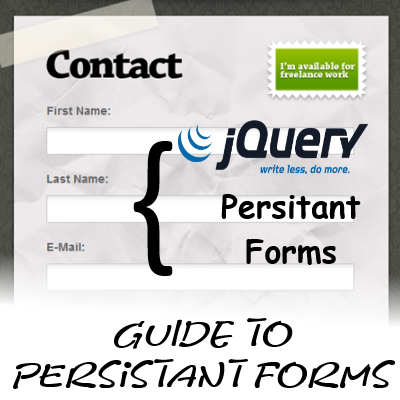# Guide to have Persistent Form data using jQueryHello Friends! Hope you all are doing good. Today I am sharing a trick that I tried in one of my projects. Here in this trick or guide we will try to have persistent form data with the help of jQuery.

The problem statement is that any times we fill form and we have.server side validations which cause the form data to be emptied out and causes the users to feel bulky in form filling. They will have to fill that form once again. Or it may happen that they have moved to some other link which will cause the form data loss.

So to solve this problem I’m here with something unique which will help in form filling.

To have Persistent Form data we will use jQuery and jQuery Cookies plugin.

The idea is to store the form data in the cookies and refill tht data if the form is called once again. And if the for submission goes successful; we will just clear the cookies.

So here it goes. We will create a one page form and include our scripts. And then when form is being navigated away; our data will be there. But for now we are only targeting on page links to navigate away. In later experiments we will handle browser navigation also.

``````<script type="text/javascript" src="jquery-1.10.2.min.js"></script>
``````

Now here is the JS function which will capture the form data and store to the cookies.

``````function saveCookie() {
\$('form').each(function(i) {
x = \$(this).attr('name');
str = \$(this).serialize();
});
}
``````

This Function will capture the data from all the forms on a webpage and save it in cookies.

Now here is the function will restore the form data

``````function restoreCookie() {
\$('form').each(function(i) {
x = \$(this).attr('name');
data = str.split('&');
\$(data).each(function(element) {
a = this.split('=');
\$('input[name="'+a+'"]').val(decodeURIComponent(a));
})
});
}
``````

This function `restoreCookie`; when called; will restore the form data.

Now here is the code which will make use of above two functions and make the scenario work.

``````\$(document).ready(function(e){
\$('a').click(function(e){
e.preventDefault();
window.location = \$(this).attr('href');
});
});
``````

So On combining all the required logic here is the complete code

``````<script>
\$('form').each(function(i) {
x = \$(this).attr('name');
str = \$(this).serialize();
});
}
\$('form').each(function(i) {
x = \$(this).attr('name');
data = str.split('&');
\$(data).each(function(element) {
a = this.split('=');
\$('input[name="'+a+'"]').val(decodeURIComponent(a));
})
});
}
function resetForm(formName){
\$(formName).reset();
}
\$('a').click(function(e){
e.preventDefault();
window.location = \$(this).attr('href');
});
});
</script>
``````

And here is the markup on which we are testing this

``````<div class="menu">
<span><a href="home.html">Home</a></span>
</div>
<label>Email</label>: <input name="email" type="text" value="" /><br />
</form>
<hr />
<form name="registerForm" method="post" action="">
<label>Name</label>: <input name="name" type="text" value="" /><br />
<label>Email</label>: <input name="email" type="text" value="" /><br />
<label>Gender</label>: <input name="gender" type="radio" value="M" /> Male  <input name="gender" type="radio" value="F" /> Female<br />